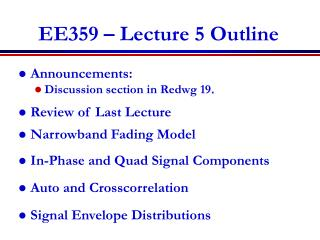DownloadDownload PresentationEE359 – Lecture 5 Outline

# EE359 – Lecture 5 Outline

Télécharger la présentation## EE359 – Lecture 5 Outline

- - - - - - - - - - - - - - - - - - - - - - - - - - - E N D - - - - - - - - - - - - - - - - - - - - - - - - - - -
##### Presentation Transcript

1. Announcements: Discussion section in Redwg 19. Review of Last Lecture Narrowband Fading Model In-Phase and Quad Signal Components Auto and Crosscorrelation Signal Envelope Distributions EE359 – Lecture 5 Outline

2. Review of Last Lecture • Model Parameters from Empirical Data • Obtain path loss model from MMSE curve fit • Obtain STD of data relative to path loss model • Random Multipath Model • Time Varying Channel Impulse Response • Random (large) number of components • -Random delays/gains/doppler on each

3. Narrowband Model • Assume delay spread maxm,n|tn(t)-tm(t)|<<1/B • Then u(t)u(t-t). • Received signal given by • No signal distortion (spreading in time) • Multipath affects complex scale factor in brackets. • Characterize scale factor by setting u(t)=ejf0

4. In-Phase and Quadratureunder CLT Approximation • In phase and quadrature signal components: • For N(t) large, rI(t) and rQ(t) jointly Gaussian by CLT (sum of large # of random vars). • Received signal characterized by its mean, autocorrelation, and cross correlation. • If jn(t) uniform, the in-phase/quad components are mean zero, indep., and stationary.

5. Auto and Cross Correlation • Assume fn~U[0,2p] • Recall that qn is the multipath arrival angle • Autocorrelation of inphase/quad signal is • Cross Correlation of inphase/quad signal is • Autocorrelation of received signal is

6. Uniform AOAs • Under uniform scattering, in phase and quad comps have no cross correlation and autocorrelation is • The PSD of received signal is Decorrelates over roughly half a wavelength Sr(f) Used to generate simulation values fc fc+fD fc-fD

7. Signal Envelope Distribution • CLT approx. leads to Rayleigh distribution (power is exponential) • When LOS component present, Ricean distribution is used • Measurements support Nakagami distribution in some environments • Similar to Ricean, but models “worse than Rayleigh” • Lends itself better to closed form BER expressions

8. Main Points • Narrowband model has in-phase and quad. comps that are zero-mean stationary Gaussian processes • Auto and cross correlation depends on AOAs of multipath • Uniform scattering makes autocorrelation of inphase and quad follow Bessel function • Signal components decorrelated over half wavelength • Cross correlation is zero (in-phase/quadrature indep.) • Fading distribution depends on environment • Rayleigh, Ricean, and Nakagami all common